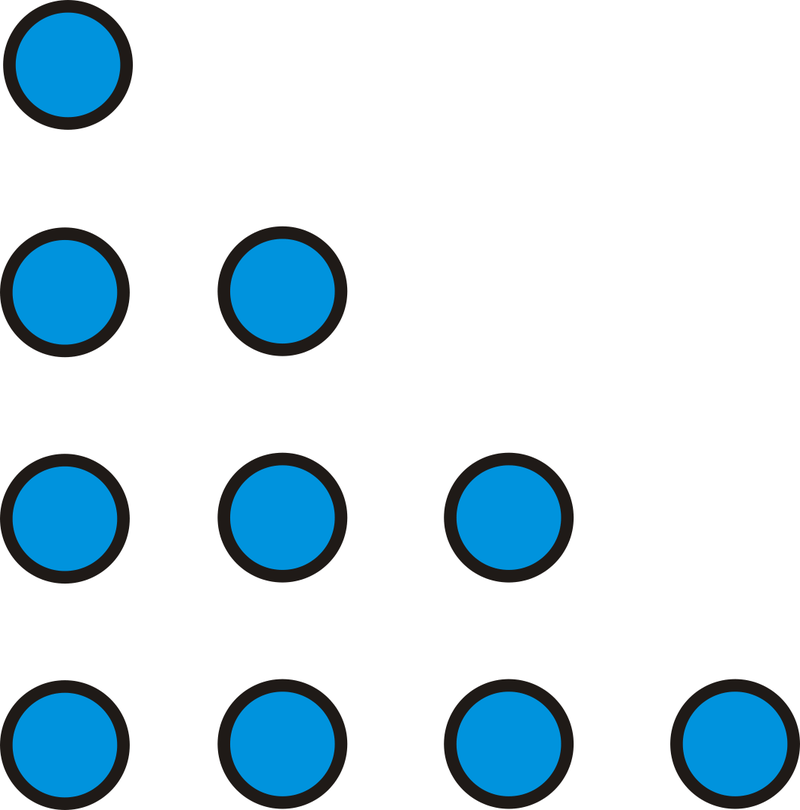# 2.5: Inductive Reasoning from Patterns

$$\newcommand{\vecs}{\overset { \rightharpoonup} {\mathbf{#1}} }$$ $$\newcommand{\vecd}{\overset{-\!-\!\rightharpoonup}{\vphantom{a}\smash {#1}}}$$$$\newcommand{\id}{\mathrm{id}}$$ $$\newcommand{\Span}{\mathrm{span}}$$ $$\newcommand{\kernel}{\mathrm{null}\,}$$ $$\newcommand{\range}{\mathrm{range}\,}$$ $$\newcommand{\RealPart}{\mathrm{Re}}$$ $$\newcommand{\ImaginaryPart}{\mathrm{Im}}$$ $$\newcommand{\Argument}{\mathrm{Arg}}$$ $$\newcommand{\norm}{\| #1 \|}$$ $$\newcommand{\inner}{\langle #1, #2 \rangle}$$ $$\newcommand{\Span}{\mathrm{span}}$$ $$\newcommand{\id}{\mathrm{id}}$$ $$\newcommand{\Span}{\mathrm{span}}$$ $$\newcommand{\kernel}{\mathrm{null}\,}$$ $$\newcommand{\range}{\mathrm{range}\,}$$ $$\newcommand{\RealPart}{\mathrm{Re}}$$ $$\newcommand{\ImaginaryPart}{\mathrm{Im}}$$ $$\newcommand{\Argument}{\mathrm{Arg}}$$ $$\newcommand{\norm}{\| #1 \|}$$ $$\newcommand{\inner}{\langle #1, #2 \rangle}$$ $$\newcommand{\Span}{\mathrm{span}}$$$$\newcommand{\AA}{\unicode[.8,0]{x212B}}$$

## Inductive Reasoning

One type of reasoning is inductive reasoning. Inductive reasoning entails making conclusions based upon examples and patterns. Visual patterns and number patterns provide good examples of inductive reasoning. Let’s look at some patterns to get a feel for what inductive reasoning is.

What if you were given a pattern of three numbers or shapes and asked to determine the sixth number or shape that fit that pattern?

Example $$\PageIndex{1}$$

A dot pattern is shown below. How many dots would there be in the $$4^{th}$$ figure? How many dots would be in the $$6^{th}$$ figure?Figure $$\PageIndex{1}$$

Solution

Draw a picture. Counting the dots, there are $$4+3+2+1=10$$ dots.Figure $$\PageIndex{2}$$

For the $$6^{th}$$ figure, we can use the same pattern, $$6+5+4+3+2+1$$. There are 21 dots in the $$6^{th}$$ figure.

Example $$\PageIndex{2}$$

How many triangles would be in the $$10^{th}$$ figure?Figure $$\PageIndex{3}$$

Solution

There would be 10 squares in the $$10^{th}$$ figure, with a triangle above and below each one. There is also a triangle on each end of the figure. That makes $$10+10+2=22$$ triangles in all.

Example $$\PageIndex{3}$$

Look at the pattern $$2, 4, 6, 8, 10, \ldots$$ What is the $$19^{th}$$ term in the pattern?

Solution

Each term is 2 more than the previous term.Figure $$\PageIndex{4}$$

You could count out the pattern until the $$19^{th}$$ term, but that could take a while. Notice that the $$1^{st}$$ term is $$2 \cdot 1$$, the 2nd term is $$2 \cdot 2$$, the 3rd term is $$2 \cdot 3$$, and so on. So, the $$19^{th}$$ term would be $$2 \cdot 19$$ or 38.

Example $$\PageIndex{4}$$

Look at the pattern: $$3, 6, 12, 24, 48, \ldots$$

What is the next term in the pattern? What is the $$10^{th}$$ term?

Solution

Each term is multiplied by 2 to get the next term.Figure $$\PageIndex{5}$$

Therefore, the next term will be $$48 \cdot 2$$ or 96.

To find the $$10^{th}$$ term, continue to multiply by 2, or $$3\cdot \underbrace{2\cdot 2\cdot 2\cdot 2\cdot 2\cdot 2\cdot 2\cdot 2 \cdot 2 }_{2^{9}}=1536$$.

Example $$\PageIndex{5}$$

Find the 8th term in the list of numbers: $$2, \dfrac{3}{4}, \dfrac{4}{9}, \dfrac{5}{16}, \dfrac{6}{25}\ldots$$

Solution

First, change 2 into a fraction, or $$\dfrac{2}{1}$$. So, the pattern is now $$\dfrac{2}{1}, \dfrac{3}{4}, \dfrac{4}{9}, \dfrac{5}{16}, \dfrac{6}{25}\ldots$$ The top is $$2, 3, 4, 5, 6$$. It increases by 1 each time, so the $$8^{th}$$ term’s numerator is 9. The denominators are the square numbers, so the $$8^{th}$$ term’s denominator is $$8^2$$ or 64. The $$8^{th}$$ term is $$\dfrac{9}{64}$$.

## Review

For questions 1-3, determine how many dots there would be in the $$4^{th}$$ and the $$10^{th}$$ pattern of each figure below.

1.Figure $$\PageIndex{6}$$
2.Figure $$\PageIndex{7}$$
3.Figure $$\PageIndex{8}$$
4. Use the pattern below to answer the questions.Figure $$\PageIndex{9}$$
1. Draw the next figure in the pattern.
2. How does the number of points in each star relate to the figure number?
5. Use the pattern below to answer the questions. All the triangles are equilateral triangles.Figure $$\PageIndex{10}$$
1. Draw the next figure in the pattern. How many triangles does it have?
2. Determine how many triangles are in the $$24^{th}$$ figure.

For questions 6-13, determine: the next three terms in the pattern.

1. $$5, 8, 11, 14, 17, \ldots$$
2. $$6, 1, -4, -9, -14, \ldots$$
3. $$2, 4, 8, 16, 32, \ldots$$
4. $$67, 56, 45, 34, 23, \ldots$$
5. $$9, -4, 6, -8, 3, \ldots$$
6. $$\dfrac{1}{2}, \dfrac{2}{3}, \dfrac{3}{4}, \dfrac{4}{5}, \dfrac{5}{6}, \ldots$$
7. $$\dfrac{2}{3}, \dfrac{4}{7}, \dfrac{6}{11},\dfrac{8}{15}, \dfrac{10}{19},\ldots$$
8. $$-1, 5, -9, 13, -17, \ldots$$

For questions 14-17, determine the next two terms and describe the pattern.

1. $$3, 6, 11, 18, 27, \ldots$$
2. $$3, 8, 15, 24, 35, \ldots$$
3. $$1, 8, 27, 64, 125, \ldots$$
4. $$1, 1, 2, 3, 5, \ldots$$

## Vocabulary

Term Definition
Inductive Reasoning Inductive reasoning is a type of reasoning where one draws conclusions from patterns and previous examples.
Equilateral Triangle An equilateral triangle is a triangle in which all three sides are the same length.

Interactive Element

Video: Inductive Reasoning

Activities: Inductive Reasoning from Patterns Discussion Questions

Study Aids: Types of Reasoning Study Guide

Practice: Inductive Reasoning from Patterns

Real World: The Science of Induction

This page titled 2.5: Inductive Reasoning from Patterns is shared under a CK-12 license and was authored, remixed, and/or curated by CK-12 Foundation via source content that was edited to the style and standards of the LibreTexts platform; a detailed edit history is available upon request.